UPSC  >  Practice Test: Pie Chart - 1

# Practice Test: Pie Chart - 1

Test Description

## 5 Questions MCQ Test Logical Reasoning (LR) and Data Interpretation (DI) | Practice Test: Pie Chart - 1

Practice Test: Pie Chart - 1 for UPSC 2022 is part of Logical Reasoning (LR) and Data Interpretation (DI) preparation. The Practice Test: Pie Chart - 1 questions and answers have been prepared according to the UPSC exam syllabus.The Practice Test: Pie Chart - 1 MCQs are made for UPSC 2022 Exam. Find important definitions, questions, notes, meanings, examples, exercises, MCQs and online tests for Practice Test: Pie Chart - 1 below.
Solutions of Practice Test: Pie Chart - 1 questions in English are available as part of our Logical Reasoning (LR) and Data Interpretation (DI) for UPSC & Practice Test: Pie Chart - 1 solutions in Hindi for Logical Reasoning (LR) and Data Interpretation (DI) course. Download more important topics, notes, lectures and mock test series for UPSC Exam by signing up for free. Attempt Practice Test: Pie Chart - 1 | 5 questions in 10 minutes | Mock test for UPSC preparation | Free important questions MCQ to study Logical Reasoning (LR) and Data Interpretation (DI) for UPSC Exam | Download free PDF with solutions
 1 Crore+ students have signed up on EduRev. Have you?
Practice Test: Pie Chart - 1 - Question 1

### The following pie-chart shows the percentage distribution of the expenditure incurred in publishing a book. Study the pie-chart and the answer the questions based on it.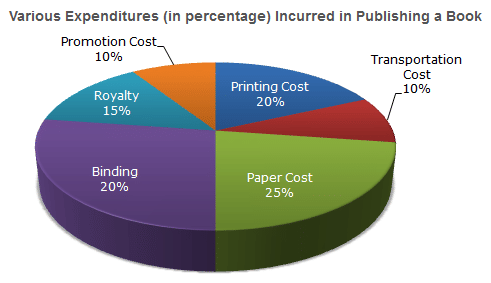If for a certain quantity of books, the publisher has to pay Rs. 30,600 as printing cost, then what will be amount of royalty to be paid for these books?

Detailed Solution for Practice Test: Pie Chart - 1 - Question 1

Let the amount of Royalty to be paid for these books be Rs. r.
Then 20:15 = 30600: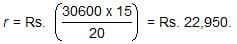Practice Test: Pie Chart - 1 - Question 2

### The following pie-chart shows the percentage distribution of the expenditure incurred in publishing a book. Study the pie-chart and the answer the questions based on it.What is the central angle of the sector corresponding to the expenditure incurred on Royalty?

Detailed Solution for Practice Test: Pie Chart - 1 - Question 2

Central angle corresponding to Royalty  = (15% of 360)0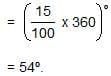Practice Test: Pie Chart - 1 - Question 3

### The following pie-chart shows the percentage distribution of the expenditure incurred in publishing a book. Study the pie-chart and the answer the questions based on it.The price of the book is marked 20% above the C.P. If the marked price of the book is Rs. 180, then what is the cost of the paper used in a single copy of the book?

Detailed Solution for Practice Test: Pie Chart - 1 - Question 3

Clearly, marked price of the book = 120% of C.P
Also  cost of paper = 25% of C.P
Let the  cost of paper for a single book be Rs. n.
Then, 120 : 25 = 180 : n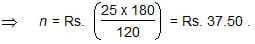Practice Test: Pie Chart - 1 - Question 4

The following pie-chart shows the percentage distribution of the expenditure incurred in publishing a book. Study the pie-chart and the answer the questions based on it.If 5500 copies are published and the transportation cost on them amounts to Rs. 82500, then what should be the selling price of the book so that the publisher can earn a profit of 25%?

Detailed Solution for Practice Test: Pie Chart - 1 - Question 4

For  the  publisher to earn a profit of  25% S.P. = 125% of C.P.
Also  Transportation Cost = 10% of C.P.
Let the S.P. of 5500 books beRs.x.
Then, 10 : 125 = 82500 : x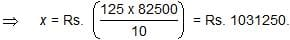∴ S.P. of  one  book = Rs.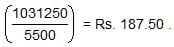Practice Test: Pie Chart - 1 - Question 5

The following pie-chart shows the percentage distribution of the expenditure incurred in publishing a book. Study the pie-chart and the answer the questions based on it.Royalty on the book is less than the printing cost by:

Detailed Solution for Practice Test: Pie Chart - 1 - Question 5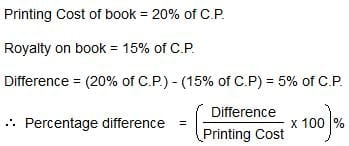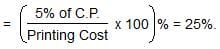## Logical Reasoning (LR) and Data Interpretation (DI)

97 videos|83 docs|119 tests
 Use Code STAYHOME200 and get INR 200 additional OFF Use Coupon Code
Information about Practice Test: Pie Chart - 1 Page
In this test you can find the Exam questions for Practice Test: Pie Chart - 1 solved & explained in the simplest way possible. Besides giving Questions and answers for Practice Test: Pie Chart - 1, EduRev gives you an ample number of Online tests for practice

## Logical Reasoning (LR) and Data Interpretation (DI)

97 videos|83 docs|119 tests

### How to Prepare for UPSC

Read our guide to prepare for UPSC which is created by Toppers & the best Teachers